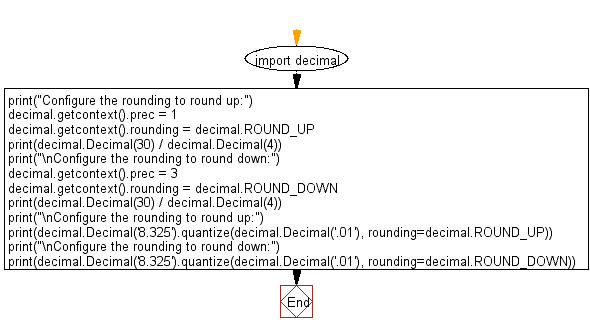﻿ Python: Configure the rounding to round up and round down a given decimal value - w3resource# Python: Configure the rounding to round up and round down a given decimal value

## Python module: Exercise-2 with Solution

Write a Python program to configure the rounding to round up and round down a given decimal value. Use decimal.Decimal

Sample Solution:

Python Code:

``````import decimal
print("Configure the rounding to round up:")
decimal.getcontext().prec = 1
decimal.getcontext().rounding = decimal.ROUND_UP
print(decimal.Decimal(30) / decimal.Decimal(4))
print("\nConfigure the rounding to round down:")
decimal.getcontext().prec = 3
decimal.getcontext().rounding = decimal.ROUND_DOWN
print(decimal.Decimal(30) / decimal.Decimal(4))
print("\nConfigure the rounding to round up:")
print(decimal.Decimal('8.325').quantize(decimal.Decimal('.01'), rounding=decimal.ROUND_UP))
print("\nConfigure the rounding to round down:")
print(decimal.Decimal('8.325').quantize(decimal.Decimal('.01'), rounding=decimal.ROUND_DOWN))
```
```

Sample Output:

```Configure the rounding to round up:
8

Configure the rounding to round down:
7.5

Configure the rounding to round up:
8.33

Configure the rounding to round down:
8.32
```

Flowchart:## Visualize Python code execution:

The following tool visualize what the computer is doing step-by-step as it executes the said program:

Python Code Editor:

Have another way to solve this solution? Contribute your code (and comments) through Disqus.

What is the difficulty level of this exercise?

Test your Python skills with w3resource's quiz

﻿

## Python: Tips of the Day

For-else construct useful when searched for something and find it:

```# For example assume that I need to search through a list and process each item until a flag item is found and
# then stop processing. If the flag item is missing then an exception needs to be raised.

for i in mylist:
if i == theflag:
break
process(i)
else:
raise ValueError("List argument missing terminal flag.")
```